Next: Heisenberg Uncertainty Principle Up: Position and Momentum Previous: Generalized Schrödinger Representation

# Momentum Representation

Consider a system with one degree of freedom, describable in terms of a coordinateand its conjugate momentum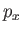, both of which have a continuous range of eigenvalues. We have seen that it is possible to represent the system in terms of the eigenkets of. This is termed the Schrödinger representation. However, it is also possible to represent the system in terms of the eigenkets of.

Consider the eigenkets ofthat belong to the eigenvalues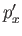. These are denoted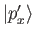. The orthogonality relation for the momentum eigenkets is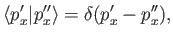(2.80)

and the corresponding completeness relation is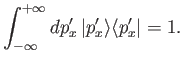(2.81)

A general state ket can be written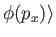(2.82)

where the standard ket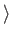satisfies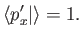(2.83)

Note that the standard ket in this representation is quite different to that in the Schrödinger representation. The momentum space wavefunction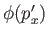satisfies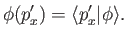(2.84)

The probability that a measurement of the momentum yields a result lying in the rangeto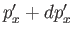is given by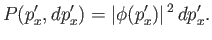(2.85)

Finally, the normalization condition for a physical momentum space wavefunction is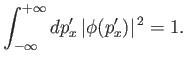(2.86)

The fundamental commutation relations (2.23)-(2.25) exhibit a particular symmetry between coordinates and their conjugate momenta. In fact, if all the coordinates are transformed into their conjugate momenta, and vice versa, and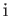is then replaced by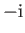, then the commutation relations are unchanged. It follows from this symmetry that we can always choose the eigenkets ofin such a manner that the coordinatecan be represented as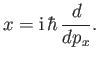(2.87)

(See Section 2.4.) This scheme is termed the momentum representation.

The previous result is easily generalized to a system with more than one degree of freedom. Suppose the system is specified bycoordinates,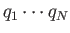, andconjugate momenta,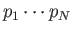. Then, in the momentum representation, the coordinates can be written as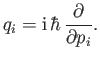(2.88)

We also have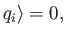(2.89)

and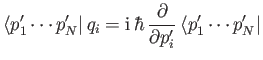(2.90)

[cf., Equation (2.78).]

Generally speaking, the momentum representation is less useful than the Schrödinger representation. The main reason for this is that the energy operators (i.e., the Hamiltonians) of most simple microscopic systems take the form of a sum of quadratic terms in the momenta (i.e., the kinetic energy) plus a complicated function of the coordinates (i.e., the potential energy). In the Schrödinger representation, the eigenvalue problem for the energy translates into a second-order partial differential equation in the coordinates, with a complicated potential function. In the momentum representation, the problem transforms into a high-order partial differential equation in the momenta, with a quadratic potential. With the mathematical tools at our disposal, we are far better able to solve the former type of differential equation than the latter.Next: Heisenberg Uncertainty Principle Up: Position and Momentum Previous: Generalized Schrödinger Representation
Richard Fitzpatrick 2016-01-22